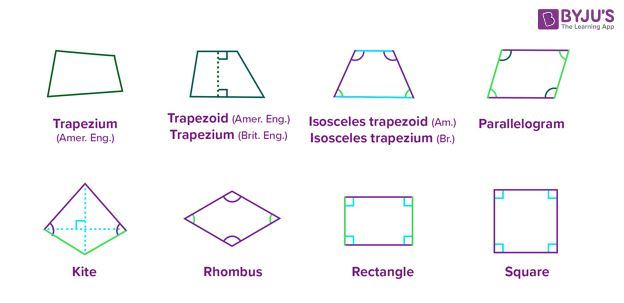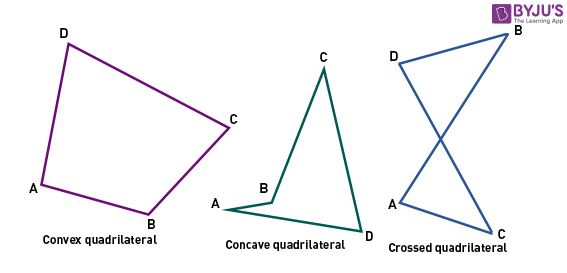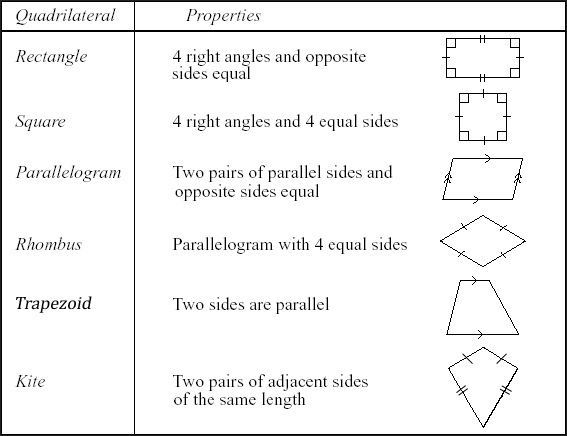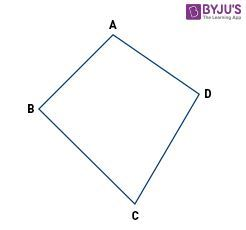In Geometry, the shapes or objects are classified based on the number of sides. The different classification of shapes are:

• Triangle ( 3-sides)
• Pentagon (5-sides)
• Hexagon (6-sides)
• Heptagon (7-sides)
• Octagon (8-sides) and so on.

A quadrilateral is a plane figure that has four sides or edges, so having four corners or vertices. Quadrilaterals will typically be of standard shapes with four sides like rectangle, square, trapezoid, and kite or irregular and uncharacterized as shown below:There are many types of quadrilaterals. As the word ‘Quad’ means four, all these types of a quadrilateral have four sides and the sum of angles of these shapes is 360 degrees.

1. Trapezium
2. Parallelogram
3. Squares
4. Rectangle
5. Rhombus
6. KiteAnother way to classify the types of quadrilaterals are:

1. Convex Quadrilaterals: Both the diagonals of a quadrilateral are completely contained within a figure.
2. Concave Quadrilaterals: At least one of the diagonals lies partly are entirely outside of the figure.

The images of these quadrilaterals are given below:A quadrilateral is a 4-sided plane figure. Below are some important properties of quadrilaterals :

• Every quadrilateral has 4 vertices, 4 angles, and 4 sides
• The total of its interior angles = 360 degrees
• A quadrilateral with four equal sides and four right angles is a square
• A quadrilateral that has opposite sides equal and measure of every angle is 90 degrees is a rectangle
• Parallelogram, trapezium, rhombus, and kite are other examples of quadrilateralsLet us understand in a better way with the help of an example:• Four sides: AB, BC, CD, and DA
• Four vertices: Points A, B, C, and D
• Four angles: ∠ABC, ∠BCD, ∠CDA, and ∠DAB
• ∠A and ∠B are adjacent angles
• ∠A and ∠C are the opposite angles
• AB and CD are the opposite sides
• AB and BC are the adjacent sides

What is the area of the quadrilateral?

Area of the quadrilateral is the total space occupied by the figure. There are different types of quadrilaterals. Let us study area formula each of them:

 Area of a Parallelogram Base x Height Area of a Rectangle Length x Width Area of a Square Side x Side Area of a Rhombus 1/2 x Diagonal 1 x Diagonal 2 OR  Base x Height Area of a Kite 1/2 x Diagonal 1 x Diagonal 2

Example 1: What is the base of a rhombus, if its area is 40 square units and the height is 8 units?
Solution:
Given,
Area = 40 square units
Height = 8 units
Area of rhombus = Base × Height
40 = Base × 8
Base = 40/8 = 5 units
Example 2: If 15 mtr and 6 mtr are diagonal lengths of a  kite, then what is its area?

Solution: Given, diagonal 1 = 15 mtr and diagonal 2 = 6 mtrSo area is simply calculated as, 1/2(15×6) = 45 square mtr.

• A quadrilateral is a trapezoid or a trapezium if 2 of its sides parallel to each other.
• A quadrilateral is a parallelogram if 2 pairs of sides parallel to each other.
• Squares and Rectangles are special types of parallelograms. Below are some special properties.– All internal angles are of “right angle” (90 degrees).– Each figure contains 4 right angles.– Sides of a square are of the same length (all sides are congruent) – Opposite sides of a rectangle are same.– Opposite sides of a rectangle and square are parallel.
• A quadrilateral is a rhombus, if
• All the sides are of equal length-Specified 2 pairs of sides are parallel to each other.
• A kite is a special sort of quadrilateral, wherever 2 pairs of adjacent sides are equal to each other.

To get more such math lessons in a simple, concise and easy to understand way. Register at BYJU’S to know more about math concepts and score well in your exams.

### What are Quadrilaterals and its types?

A quadrilateral can be defined as a plane figure having 4 sides. There are mainly 6 types of quadrilaterals which are:

• Trapezium
• Parallelogram
• Rectangle
• Rhombus
• Square
• Kite

Apart from these 6 types, a quadrilateral can also be classified as: